Deep Learning(1) Introduction of Deep Learning
2023-11-18 21:46:51

# Machine Learning

Looking for Function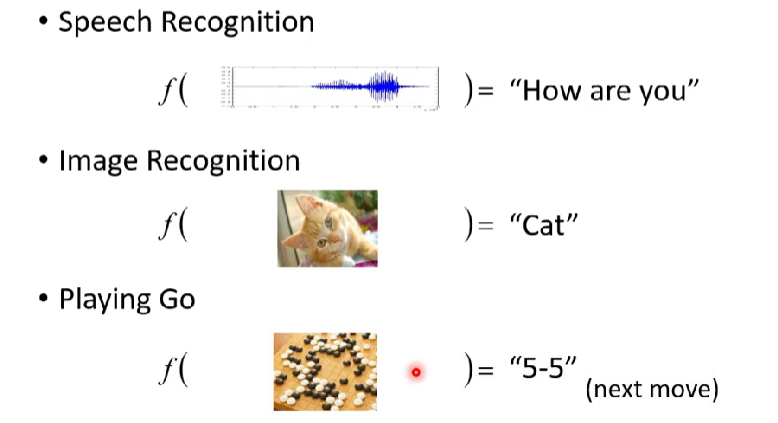Different types of Functions：

Regression(回归): 函数输出一个数值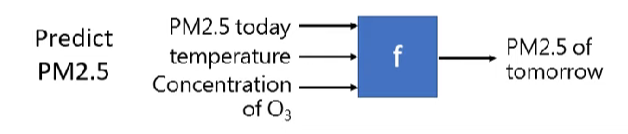Classification(分类): 函数从设定好的选项选择出正确的一个, 即分类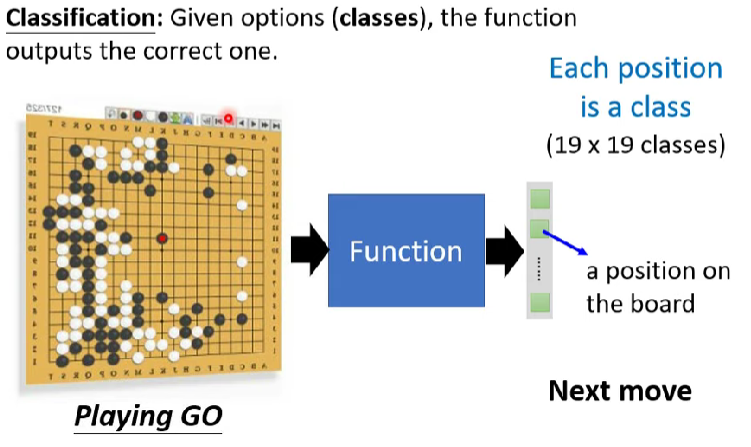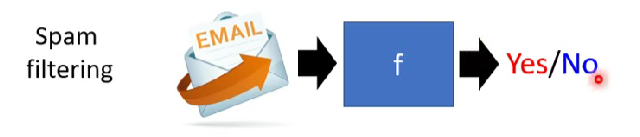Structured Learning: 产生一些有结构的物件(让机器学会创造)

• create something with structure(image, document)

## 1 A Case Study

### Function with Unknown Parameters

• $y$: 明日的观看人数

• $x_1$: 今日的观看人数 (feature)

• $w, b$: 未知参数 (weight, bias)

### Define Loss from Training Data

Loss 也是一个函数 $L(b, w)$, 输入是 Model 里的参数

• 用来评价 $b, w$ 取值的好坏
• how good a set of values is

• 从训练集中已有的数据计算Loss
• 真实值为 label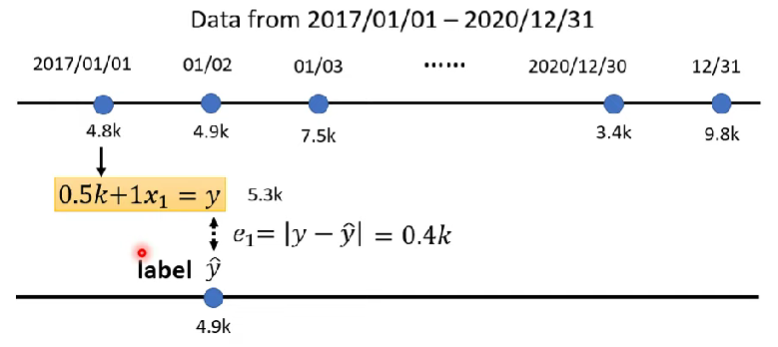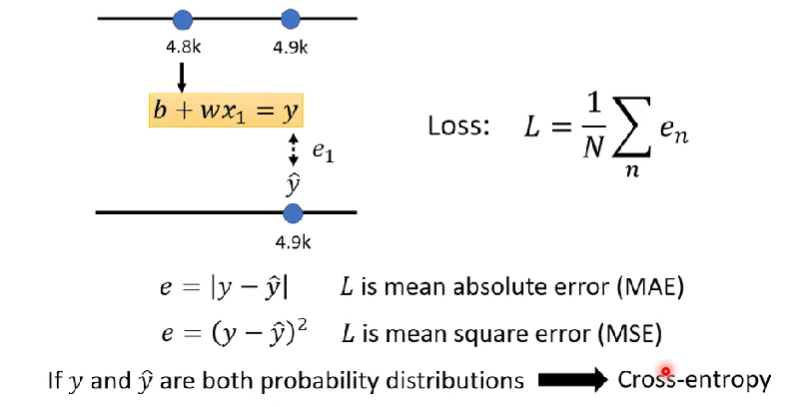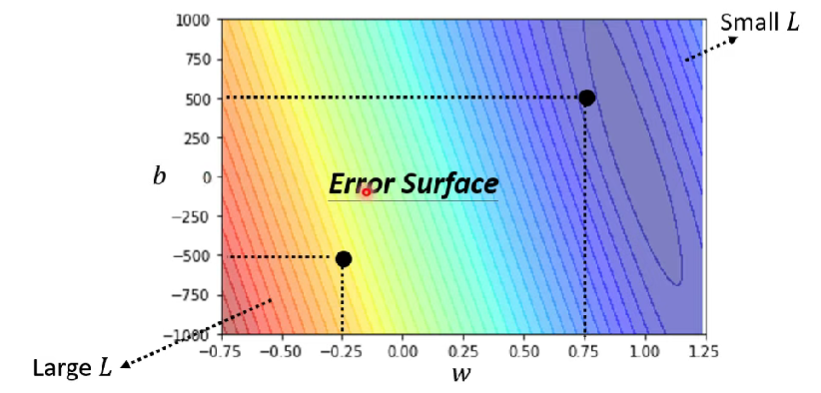### Optimization

$w^{\ast}, b^{\ast} = arg \min\limits_{w, b}L$

• 找到能让损失函数值最小的参数

1. 随机选取初始值 $w_0$

2. 计算在 $w=w_0$ 的时候, $w$ 这个参数对 $L$ 的微分是多少, 即斜率

3. 不断根据微分(梯度)的方向，改变参数的值, 斜率为负就增加 $w$, 反之减少

• 改变的大小取决于：

• 斜率的大小

• 学习率的大小**(超参数)**

• hyperparameters: 使用者自己决定的值
• 什么时候停下来？

• 自己设置上限（超参数）
• 理想情况：微分值为0(极小值点)，不会再更新 -> 有可能陷入局部最小值，不能找到全局最小值
• 事实上：局部最小值不是真正的问题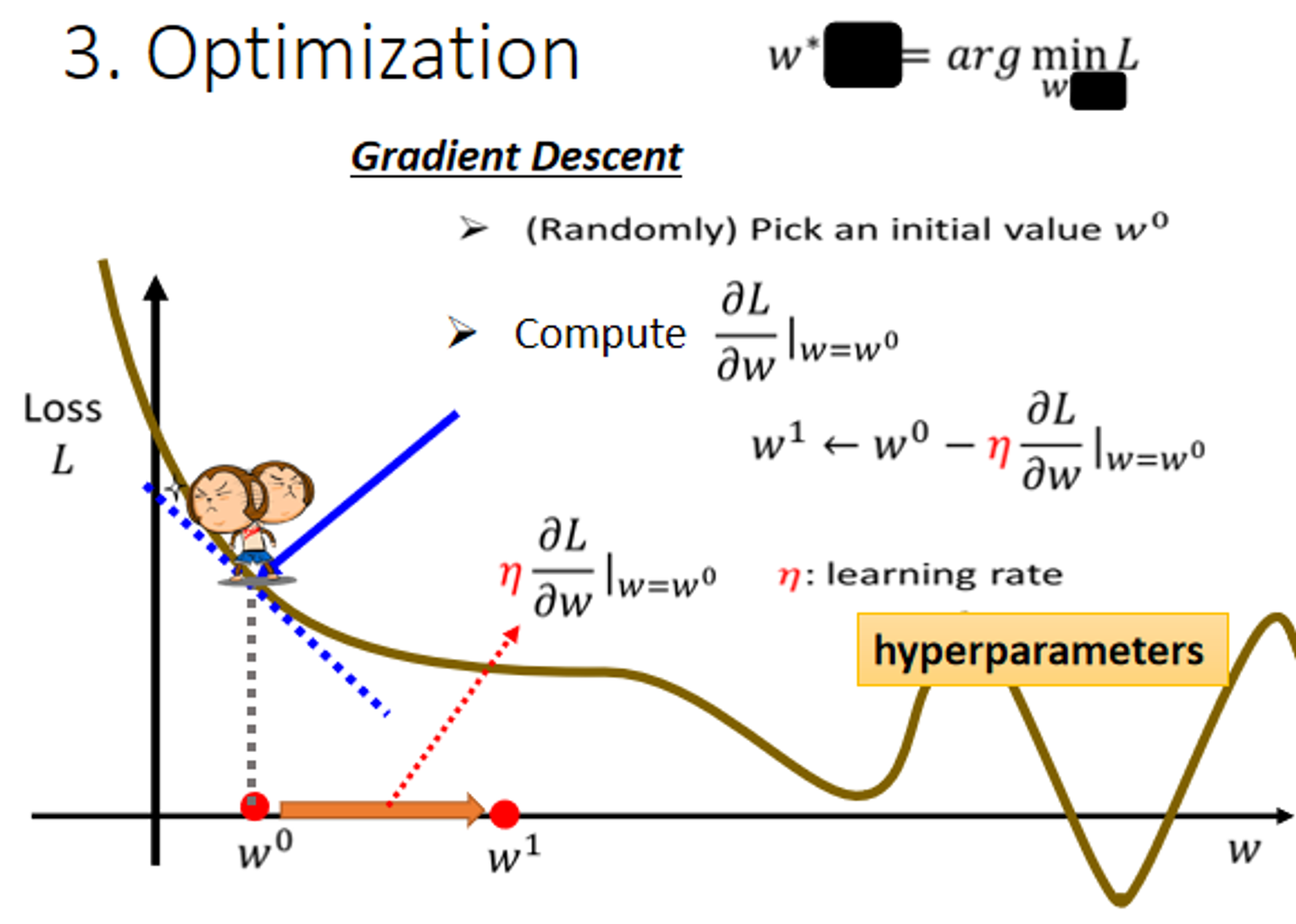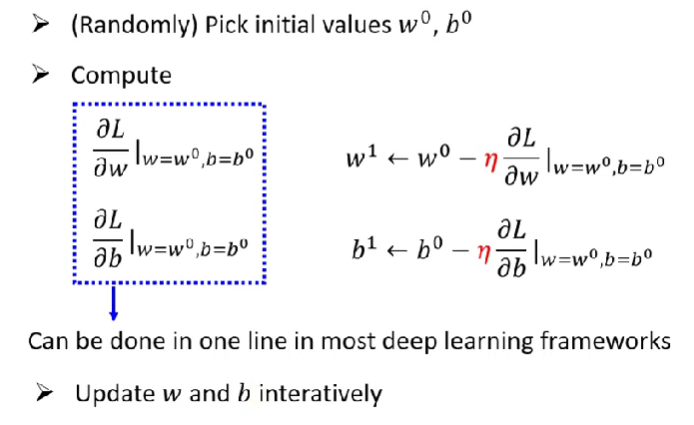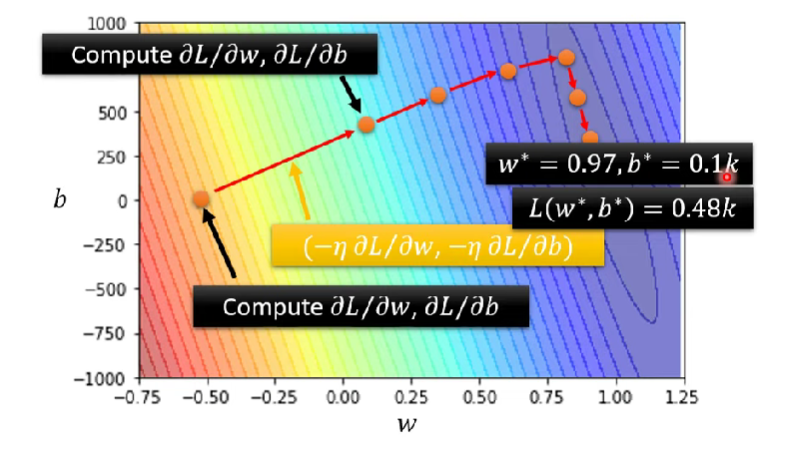## 2 Linear Model(线性模型)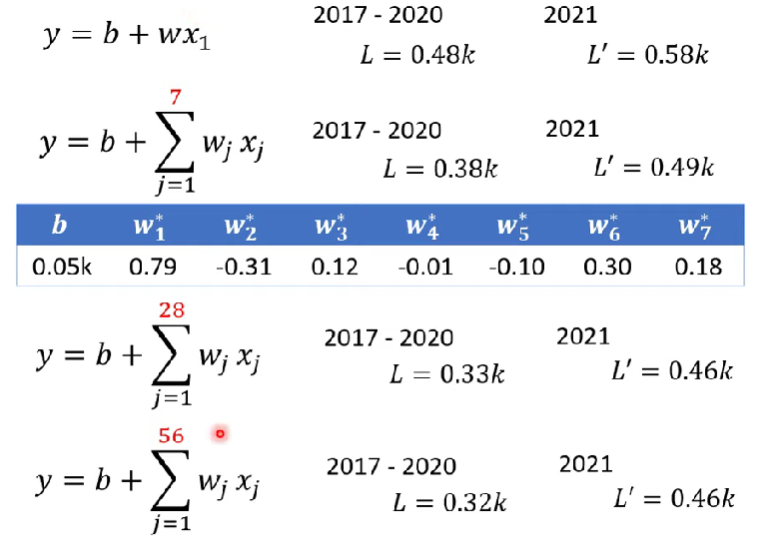### 2.1 Piecewise Linear Curve(分段线性曲线)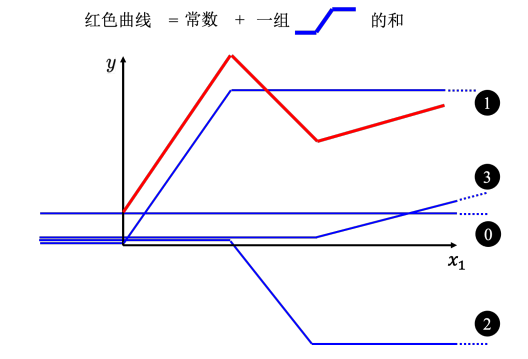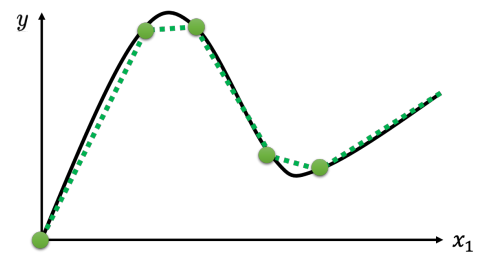$$y=c\frac{1}{1+e^{-(b+wx_1)}}$$

$$y=\textcolor{red}{c}\ sigmoid(b+wx_1)$$2023-11-18 21:46:51